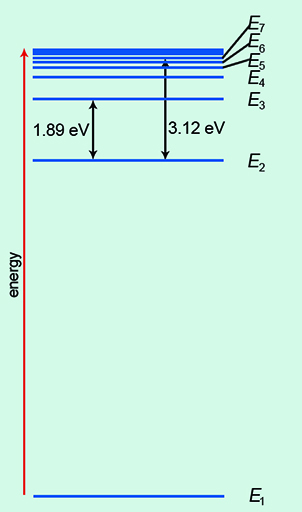Science, Maths & Technology

### Become an OU studentMicrogravity: living on the International Space Station

Start this free course now. Just create an account and sign in. Enrol and complete the course for a free statement of participation or digital badge if available.

# 4 Sodium D-lines and the hydrogen atom

Energy levels are demonstrated in the context of a sodium lamp in Video 3. Watch it now and then do Activity 4.Video 3 Sodium D-lines.
Interactive feature not available in single page view (see it in standard view).

## Activity 4 Energy levels

Timing: Allow approximately 15 minutes

For each question, choose the one correct answer from the options given below.

1. The sodium lamp has the following number of lines:

a.

zero

b.

1

c.

2

d.

5

e.

10

Active content not displayed. This content requires JavaScript to be enabled.
Interactive feature not available in single page view (see it in standard view).
Active content not displayed. This content requires JavaScript to be enabled.
Interactive feature not available in single page view (see it in standard view).

Video 3 introduced the concept of energy levels. These can be thought of as the rungs on a ladder (Figure 5).Figure 5 The analogy of rungs on a ladder for energy levels.

Figure 5 represents the simplest atom, hydrogen. This atom has one electron ‘in orbit’ around the nucleus (labelled as the blue circle with the letter ‘e’). The nucleus of a hydrogen atom contains just one proton (labelled as the red circle with the letter ‘p’). The rungs on the ladder represent discrete and separate energy levels. In the ‘ground state’, the electron would be on the first rung of the ladder. This state is given the value n = 1. (Please note that this is not the same ‘n’ as in the diffraction equation). If you give the electron exactly the right amount of energy, it will then move up to the next rung of the ladder. Here it would be the second rung where n = 2. You can test your understanding of this in the next activity.

## Activity 5 Energy levels in a hydrogen atom (ladder analogy)

Timing: Allow approximately 15 minutes

Choose the correct answer from the options to complete the following statements.

1. The energy state of the electron in Figure 5 is:

a.

n = 5

b.

n = 4

c.

n = 3

d.

n = 2

e.

n = 1

2. The highest energy state of the electron in Figure 5 would be:

a.

n = 3

b.

n = 4

c.

n = 5

d.

n = 6

e.

n = 7

Of course, the real picture is more complicated than a simple ladder! In Figure 6, the ground state of energy is labelled E1. As you move up the energy levels, they bunch closer together. The electrons can transition, or move, between each energy level. Indeed, there are numerous permutations.Figure 6 Energy levels of a hydrogen atom.

You can see that the energy units of eV, or electronvolts, are used here. At this size in the quantum world, the usual unit of energy, the joule, is much too big.

Now complete this section on energy levels by doing Activity 6.

## Activity 6 Energy levels in a hydrogen atom

Timing: Allow approximately 10 minutes

For each question, choose the one correct answer from the options given.

1. How many energy levels are shown in Figure 5?

a.

7

b.

6

c.

5

d.

3

e.

4

2. The 1.89 eV transition is between the following energy levels.

a.

E1 and E2

b.

E1 and E3

c.

E2 and E3

d.

E3 and E4

e.

E4 and E5

3. The 3.12 eV transition is between the following energy levels.

a.

E1 and E7

b.

E2 and E7

c.

E3 and E7

d.

E4 and E7

e.

E5 and E7